Next: Incorporation of prior knowledge Up: Maximum Likelihood estimation Previous: Model order selection

## Estimation of the unmixing matrix

Recall from equation 5 that in order to estimate the mixing matrix and the sources, we need to optimise an orthogonal rotation matrix in the space of whitened observations: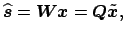(11)

where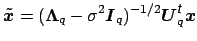denotes the spatially whitened data.

In order to choose a technique for the unmixing step note that all previous results have highlighted the importance of non-Gaussianity of the source distributions: the split into a non-Gaussian part plus additive Gaussian noise is at the heart of the uniqueness results. Also, the estimation of the intrinsic dimensionality is based on the identification of eigenvectors of the data covariance matrix that violate the sphericity assumption of the isotropic Gaussian noise model. Consistent with this, we will estimate the unmixing matrix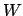based on the principle of non-Gaussianity. [Hyvärinen et al., 2001] have presented an elegant fixed point algorithm that uses approximations to neg-entropy in order to optimise for non-Gaussian source distributions and give a clear account of the relation between this approach to statistical independence. In brief, the individual sources are obtained by projecting the data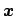onto the individual rows of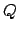, i.e. the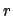th source is estimated as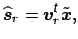where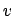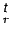denotes theth row of. In order to optimise for non-Gaussian source estimates, [Hyvärinen et al., 2001] propose the following contrast function: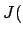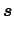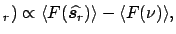(12)

wheredenotes a standardised Gaussian variable and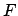is a general non-quadratic function that combines the high-order cumulants of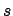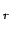in order to approximate the 'true' neg-entropy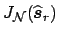. From equation 12, the vectoris optimised to maximise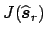using an approximative Newton method. This finally leads to the following fixed point iteration scheme: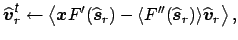(13)

where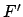denotes the derivative of. This is followed by a re- normalisation step such thatis of unit length. A proof of convergence and discussion about the choice of the non- linear function can be found in [Hyvärinen et al., 2001]. In order to estimate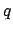sources, this estimation is simply performedtimes under the constraint that the vectors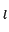are mutually orthogonal. The constraint on the norm and the mutual orthogonality assure that these vectors actually form an orthogonal rotation matrix. Thus, estimation of the sources is carried out under the assumption that all marginal distributions of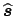have maximally non-Gaussian distribution.

The choice of the nonlinear function is domain specific and in our case will be strongly linked to the inferential steps that are being performed after IC estimation (see section 4 below).Next: Incorporation of prior knowledge Up: Maximum Likelihood estimation Previous: Model order selection
Christian F. Beckmann 2003-08-05